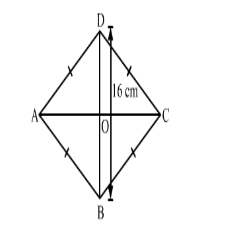# The length of each side of a rhombus is 10 cm and one if its diagonals is of length 16 cm.

Question:

The length of each side of a rhombus is 10 cm and one if its diagonals is of length 16 cm. The length of the other diagonal is
(a) 13 cm
(b) 12 cm

(c) $2 \sqrt{39} \mathrm{~cm}$

(d) $6 \mathrm{~cm}$

Solution:

(b) 12 cm

Explanation:Let $A B C D$ be the rhombus.

$\therefore A B=B C=C D=D A=10 \mathrm{~cm}$

Let AC and BD be the diagonals of the rhombus.
Let
AC be x and BD be 16 cm and O be the intersection point of the diagonals.

We know that the diagonals of a rhombus are perpendicular bisectors of each other.

$\therefore \triangle A O B$ is a right angle triangle in which $O A=A C \div 2=x \div 2$ and $O B=B D \div 2=16 \div 2=8 \mathrm{~cm} .$

Now, $A B^{2}=O A^{2}+O B^{2}$      [Pythagoras theorem]

$\Rightarrow 10^{2}=\left(\frac{x}{2}\right)^{2}+8^{2}$

$\Rightarrow\left(\frac{x}{2}\right)^{2}=36=6^{2}$

$\Rightarrow x=2 \times 6=12 \mathrm{~cm}$